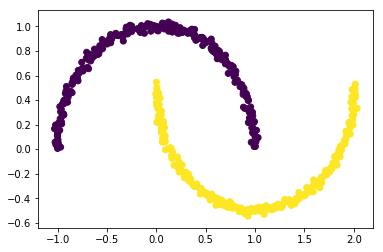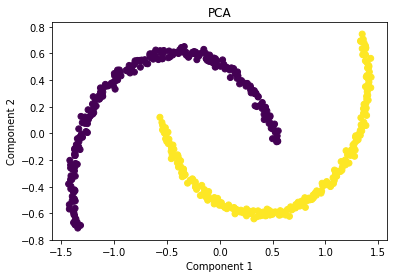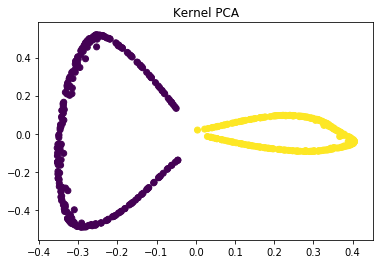# ML | Introduction to Kernel PCA

PRINCIPAL COMPONENT ANALYSIS: is a tool which is used to reduce the dimension of the data. It allows us to reduce the dimension of the data without much loss of information. PCA reduces the dimension by finding a few orthogonal linear combinations (principal components) of the original variables with the largest variance.
The first principal component captures most of the variance in the data. The second principal component is orthogonal to the first principal component and captures the remaining variance, which is left of first principal component and so on. There are as many principal components as the number of original variables.
These principal components are uncorrelated and are ordered in such a way that the first several principal components explain most of the variance of the original data. To learn more about PCA you can read the article Principal Component Analysis

KERNEL PCA:

PCA is a linear method. That is it can only be applied to datasets which are linearly separable. It does an excellent job for datasets, which are linearly separable. But, if we use it to non-linear datasets, we might get a result which may not be the optimal dimensionality reduction. Kernel PCA uses a kernel function to project dataset into a higher dimensional feature space, where it is linearly separable. It is similar to the idea of Support Vector Machines.

There are various kernel methods like linear, polynomial, and gaussian.

Code: Create a dataset which is nonlinear and then apply PCA on the dataset.

 `import` `matplotlib.pyplot as plt ` `from` `sklearn.datasets ``import` `make_moons ` ` `  `X, y ``=` `make_moons(n_samples ``=` `500``, noise ``=` `0.02``, random_state ``=` `417``) ` ` `  `plt.scatter(X[:, ``0``], X[:, ``1``], c ``=` `y) ` `plt.show() `Code: Let’s apply PCA on this dataset

 `from` `sklearn.decomposition ``import` `PCA ` `pca ``=` `PCA(n_components ``=` `2``) ` `X_pca ``=` `pca.fit_transform(X) ` `  `  `plt.title(``"PCA"``) ` `plt.scatter(X_pca[:, ``0``], X_pca[:, ``1``], c ``=` `y) ` `plt.xlabel(``"Component 1"``) ` `plt.ylabel(``"Component 2"``) ` `plt.show() `As you can see PCA failed to distinguish the two classes.

Code: Applying kernel PCA on this dataset with RBF kernel with a gamma value of 15.

 `from` `sklearn.decomposition ``import` `KernelPCA ` `kpca ``=` `KernelPCA(kernel ``=``'rbf'``, gamma ``=` `15``) ` `X_kpca ``=` `kpca.fit_transform(X) ` ` `  `plt.title(``"Kernel PCA"``) ` `plt.scatter(X_kpca[:, ``0``], X_kpca[:, ``1``], c ``=` `y) ` `plt.show() `In the kernel space the two classes are linearly separable. Kernel PCA uses a kernel function to project the dataset into a higher-dimensional space, where it is linearly separable.
Finally, we applied the kernel PCA to a non-linear dataset using scikit-learn.

My Personal Notes arrow_drop_upCheck out this Author's contributed articles.

If you like GeeksforGeeks and would like to contribute, you can also write an article using contribute.geeksforgeeks.org or mail your article to contribute@geeksforgeeks.org. See your article appearing on the GeeksforGeeks main page and help other Geeks.

Please Improve this article if you find anything incorrect by clicking on the "Improve Article" button below.

Article Tags :
Practice Tags :

Be the First to upvote.

Please write to us at contribute@geeksforgeeks.org to report any issue with the above content.